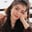Related Tags

matplotlib
communitycreator

# What is a quiver plot in Matplotlib?Fouzia Bashir

## Quiver plot

A quiver or vector plot is used to plot 2-dimensional vector lines as arrows. This type of plot is useful where magnitude and direction are required. For instance, a quiver plot is used to visualize the electric field intensity in any direction. It can also be used to visualize the electrical potential in electrical engineering.

Quiver plot

The quiver() method is used to generate a quiver plot in Matplotlib. It creates a grid of X-Y values and two sets of U-V directional components.

### Syntax

matplotlib.pyplot.quiver(X, Y, U, V, [C], **kw)

### Parameters

It takes the following argument values:

• X: It can be either a 1D or 2D array along the x-axis. It is the value or set of the x coordinate of the arrow location.
• Y: It can be either a 1D or 2D array along the y-axis. It is the value or set of the y coordinate of the arrow location.
• U: x-component of the arrow vector.
• V: y-component of the arrow vector.
• [C]: Optional argument used to specify arrow colors.
• **kw: Additional keyword arguments.

### Return value

It returns an instance of matplotlib.quiver.Quiver module.

### Explanation

In the code snippet below, we are going to plot a single arrow based quiver graph. The quiver() method will take x=1, y=2, u=[1,0], and v=[1,-1] as arguments and print two arrows in different directions.

# including numpy and pyplot from matplotlib module
import numpy as np
import matplotlib.pyplot as plt
# Creating quiver plot arguments
x = 1
y = 2
u = [1, 0]
v = [1, -1]
# Creating plot
plt.quiver(x, y, u, v)
# Show plot
plt.show()
plt.title('The quiver() Example')
# It is used to display results in the plot format
plt.savefig('output/graph.png')
• Lines 2–3: We import the required libraries.
• Lines 5–8: We define random points x=1, y=2, u=[1,0], and v=[1,-1].
• Line 10: We invoke the quiver() function from the Pyplot module to generate a double arrowed quiver plot.
• Lines 12–15: The show() method will demonstrate the generated plot on the console, title('The quiver() Example') will add the figure title on the generated output graph, and the savefig() method will save the output as graph.png temporarily.

RELATED TAGS

matplotlib
communitycreator

CONTRIBUTORFouzia Bashir
RELATED COURSES

View all Courses

Keep Exploring

Learn in-demand tech skills in half the time